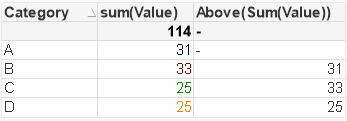# QlikView App Dev

Discussion Board for collaboration related to QlikView App Development.

Announcements
cancel
Showing results for
Did you mean:Specialist

## Conditional colouring

I should know the answer to this, but I seem to be having a problem with a solution, basically I have a straight table of only 3 rows, and I want to conditionally colour code (text) the 2nd and 3rd row field 1 based on how they compare to Row 1 field 1 e.g.

Row 1 field 1 stays black,

If Row 2 field 1 is greater than Row 1 field 1 then colour is red , if same then orange else green.

There is a single dimension that is used e.g. Category , so in effect I am testing the values across the dimension rows.

I don' want to write the calculations i.e. the SUM's etc into the colour condition as this becomes clumsy.

Anyone tried this before ? I need an AHA momentThanks

Paul

1 Solution

Accepted SolutionsSpecialist
Author

Ok here is my solution after a quick walk around the block in the sunshineif([Avg Cost] < above([Avg Cost],if(RowNo()=3,2,1)),Green(128),

if([Avg Cost] = above([Avg Cost],if(RowNo()=3,2,1)),Yellow(128)

,if([Avg Cost] > above([Avg Cost],if(RowNo()=3,2,1)),Red(128)

,Black()

)))

So Note, My table has only 3 rows and will always be 3 rows.

9 RepliesContributor II

G'day Paul,

I put a flag in the load script and did the comparison there

...

IF( 'row' > PREVIOUS ('row'), 1,0) as myFlag,

....

From

...

order by dimension

then in the chart I put

IF (myFlag = 1, red(), yellow())

cheers,Not applicable

Write this expression in your Background color

if(Amount = Above(Amount), Yellow(),

if(Amount > above(Amount), Red(), Green()))Specialist
Author

No sorry, my point perhaps is not clear, The First Row in the table is what I am comparing my

ROW 2 and ROW 3 against .Specialist
Author

Ok here is my solution after a quick walk around the block in the sunshineif([Avg Cost] < above([Avg Cost],if(RowNo()=3,2,1)),Green(128),

if([Avg Cost] = above([Avg Cost],if(RowNo()=3,2,1)),Yellow(128)

,if([Avg Cost] > above([Avg Cost],if(RowNo()=3,2,1)),Red(128)

,Black()

)))

So Note, My table has only 3 rows and will always be 3 rows.Not applicable

Got It

if(Amount = above(Amount, RowNo()-1), Yellow(),

if(Amount > above(Amount, RowNo()-1), Red(), Green()))Specialist III

Missing Manual - FieldValue

This can also reduce effort , check if required.Creator III

Hi Paul,

In Text Color option:You can add an expression like this:

If(IsNull(Above(sum(Value))) = 0,

if(sum(Value) > Above(sum(Value)), red(),

if(sum(Value) = Above(Sum(Value)), RGB(255,128,0), green())), Black())

This expression evaluate the previous value, if it's not null it will check if the current value is greater or equal than the previous and apply the corresponding color code, otherwise font will be black. Here is an example of the output of this function:Hope it helps.

- KarlaNot applicable

Yes Agnivesh. That makes it even more simple.

if(Amount = FieldValue('Amount',1), Yellow(),

if(Amount > FieldValue('Amount',1), Red(), Green()))Specialist
Author

Thanks for all the ideas, the one I posted is working the way I want it